## Fermi Level in Extrinsic Semiconductor:

The equation for f(E) specifying the fraction of all states at energy E (electron volts) occupied under conditions of thermal equilibrium is called the Fermi-Dirac probability function and is given as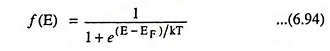where k is Boltzmann constant in eV/K, T is temperature in K and EF is the Fermi level or characteristic energy for crystal in eV.

Concentrations of free electrons n and concentration of holes p are given as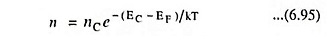and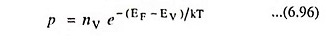where nC is the number of electrons in conduction band, nV is the number of electrons in the valence band, EC is the lowest energy in the conduction band in eV and EV is the maximum energy of valence band in eV.

The only parameter that changes with the addition of impurity in Eqs. (6.95) and (6.96) is the Fermi level.

Fermi Level in N-type Extrinsic Semiconductor: In case of an intrinsic semiconductor, EF lies in the middle of the energy gap, indicating equal concentrations of free electrons and holes.

When a donor type impurity is added to the crystal, then, at a given temperature and assuming all donor atoms are ionized, the first ND states in the conduction band will be filled. Hence it will be more difficult for the electrons from the valence band to bridge the energy gap by the thermal agitation. Consequently the number of electron-hole pairs thermally generated for that temperature will be reduced. Since the Fermi Level in Extrinsic Semiconductor is a measure of the probability of occupancy of the allowed energy states, it is clear that EF must be more closer to the conduction band to indicate that many of the energy states in that band are filled by the donor electrons, and fewer holes exist in the valence band. This situation is illustrated in Fig. 6.29, for an N-type extrinsic semiconductor.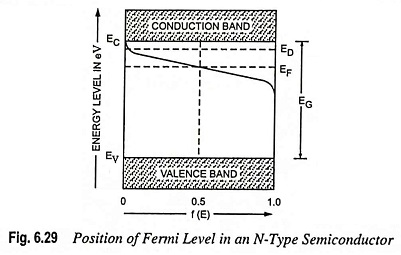The same kind of argument leads to the conclusion that EF must move from the centre of the forbidden gap closer to the valence band for a P-type extrinsic semiconductor.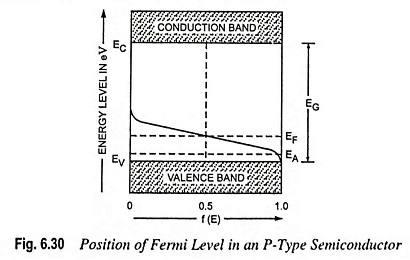Exact position of the Fermi level in an N-type extrinsic semiconductor can be determined by substituting n = ND in Eq. (6.95) and thus we have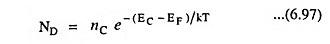Taking logarithm we have,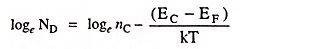Solving the above equation we have,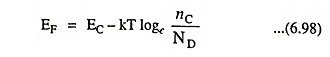Similarly for the P-type extrinsic semiconductor,

Scroll to Top